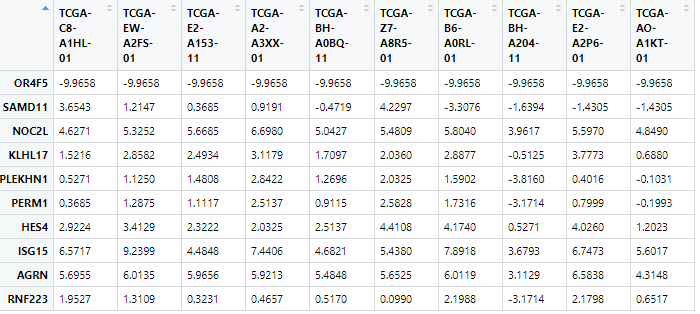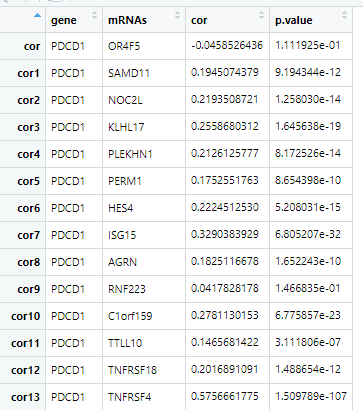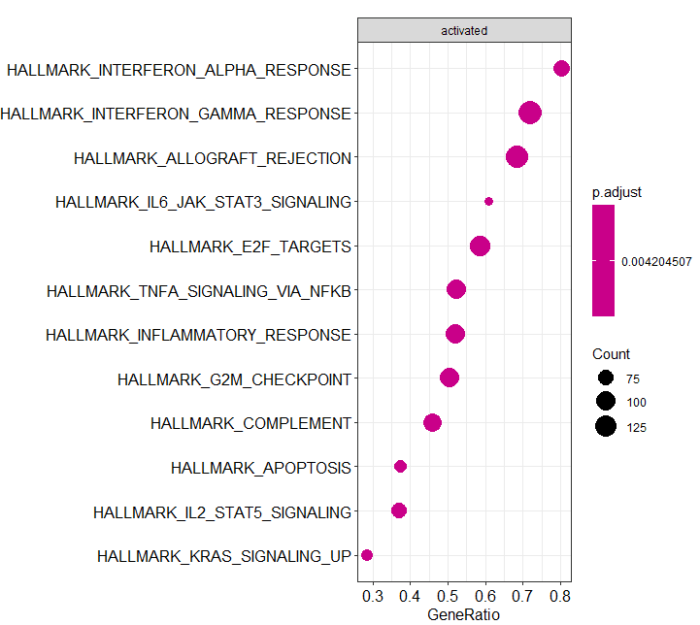# 基于单基因批量相关性分析的GSEA

1.已经确定研究的基因，但是想探索他潜在的功能，可以通过跟这个基因表达最相关的基因来反推他的功能，这种方法在英语中称为guilt of association，协同犯罪。

2.我们的注释方法依赖于TCGA大样本，既然他可以注释基因，那么任何跟肿瘤相关的基因都可以被注释，包括长链非编码RNA。

GSEA需要的gene set是现成的没有问题，但是genelist没有，这里我们可以把所有基因跟单个基因的相关性系数当做LogFC，有正有负，就解决了geneList的问题。这个想法不是我的，是我的一个学员的，不过他要解决的是microRNA把基因的问题。

1.首先加载数据

```load(file = "BRCA_mRNA_exprSet.Rdata")
exprSet <- mRNA_exprSet
test <- exprSet[1:10,1:10]```2.写一个函数批量计算相关性

```batch_cor <- function(gene){
y <- as.numeric(exprSet[gene,])
rownames <- rownames(exprSet)
do.call(rbind,future_lapply(rownames, function(x){
dd  <- cor.test(as.numeric(exprSet[x,]),y,type="spearman")
data.frame(gene=gene,mRNAs=x,cor=dd\$estimate,p.value=dd\$p.value )
}))
}```

3.并行化运行函数

```library(future.apply)
plan(multiprocess)
system.time(dd <- batch_cor("PDCD1"))```4.制作genelist gene

```gene <- dd\$mRNAs
## 转换
library(clusterProfiler)
gene = bitr(gene, fromType="SYMBOL", toType="ENTREZID", OrgDb="org.Hs.eg.db")
## 去重
gene <- dplyr::distinct(gene,SYMBOL,.keep_all=TRUE)

gene_df <- data.frame(logFC=dd\$cor,
SYMBOL = dd\$mRNAs)
gene_df <- merge(gene_df,gene,by="SYMBOL")

## geneList 三部曲
## 1.获取基因logFC
geneList <- gene_df\$logFC
## 2.命名
names(geneList) = gene_df\$ENTREZID
## 3.排序很重要
geneList = sort(geneList, decreasing = TRUE)```

5.运行GSEA分析

```library(clusterProfiler)
## 读入hallmarks gene set，从哪来？
# 需要网络
y <- GSEA(geneList,TERM2GENE =hallmarks)```

```### 看整体分布
library(ggplot2)
dotplot(y,showCategory=12,split=".sign") facet_grid(~.sign)```6.特定通路作图

yd <- data.frame(y)
library(enrichplot)
gseaplot2(y,"HALLMARK_INTERFERON_ALPHA_RESPONSE",color = "red",pvalue_table = T)

PCDC1跟阿拉法干扰素正相关，这个事情没什么好说的吧。

• 本文由 发表
• 转载请务必保留本文链接：https://www.plob.org/article/26317.html